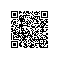# [从C到C++] 1.10 C++ 异常处理（try和catch）

C++新增throw关键字用于抛出异常新增catch关键字用于捕获异常新增try关键字尝试捕获异常。通常将尝试捕获的语句放在 try{ } 程序块中，而将异常处理语句置于 catch{ } 语句块中。

throw 表达式;

try
{
//可能抛出异常的语句
}
catch (异常类型1)
{
//异常类型1的处理程序
}
catch (异常类型2)
{
//异常类型2的处理程序
}
// ……
catch (异常类型n)
{
//异常类型n的处理程序
}


[例1] C++异常处理示例：


#include<iostream>
using namespace std;
enum index{underflow, overflow};
int array_index(int *A, int n, int index);
int main()
{
int *A = new int;
for(int i=0; i<10; i++)
A[i] = i;
try
{
cout<<array_index(A,10,5)<<endl;
cout<<array_index(A,10,-1)<<endl;
cout<<array_index(A,10,15)<<endl;
}
catch(index e)
{
if(e == underflow)
{
cout<<"index underflow!"<<endl;
exit(-1);
}
if(e == overflow)
{
cout<<"index overflow!"<<endl;
exit(-1);
}
}
return 0;
}
int array_index(int *A, int n, int index)
{
if(index < 0) throw underflow;
if(index > n-1) throw overflow;
return A[index];
}

[例2] 捕获new、new[] 抛出的异常：

int * p;
try
{
p = new int;
}
{
cerr<<"allocate failure!"<<endl;
exit(-1);
}使用钉钉扫一扫加入圈子
+ 订阅